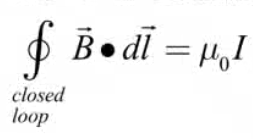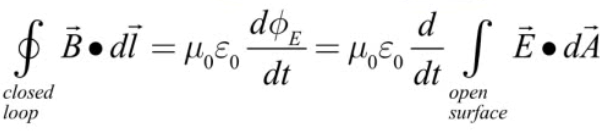# Maxwell's Equations# Revisiting Ampere's Law

• Ampere's Law as written allows us to calculate the magnetic field due to an electric current.

• We also know that a changing electric field produces a magnetic field

• Combine effects of electric current and changing E field on magnetic field to obtain a more complete version of Ampere's Law

• Contribution due to the penetrating current is known a conduction current.• Contribution due to changing electric field is known as the displacement current• Final Ampere's Law